# Edexcel A Level Chemistry:复习笔记5.3.2 Born-Haber Cycles

### Born-Haber Cycles

• A Born-Haber cycle is a specific application of Hess’ Law for ionic compounds and enable us to calculate lattice enthalpy which cannot be found by experiment
• The basic principle of drawing the cycle is to construct a diagram in which energy increases going up the diagram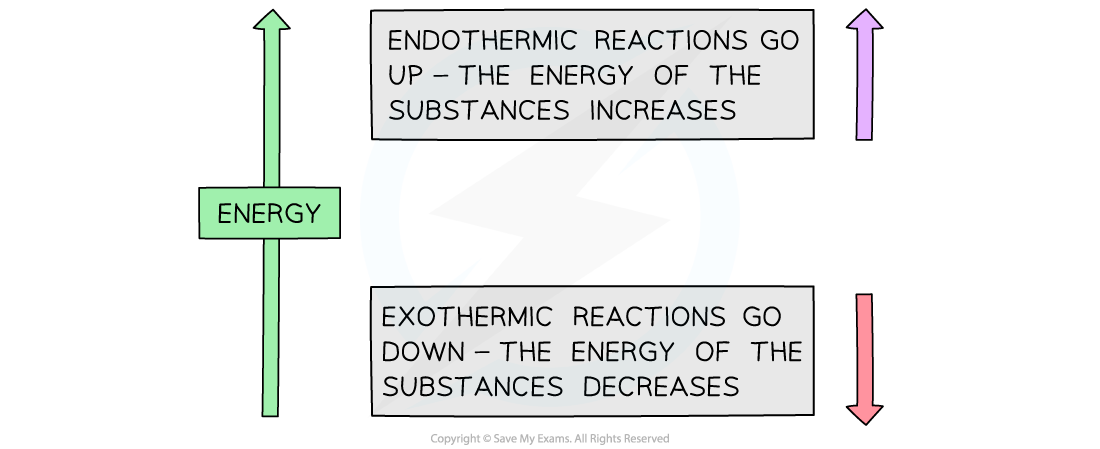The basic principle of a Born-Haber cycle

• The cycle shows all the steps needed to turn atoms into gaseous ions and from gaseous ions into the ionic lattice
• The alternative route to the ionic lattice begins from the enthalpy of formation of the elements in their standard states

#### Drawing the cycle for sodium chloride

• A good starting point is to draw the elements with their state symbols about a third of the way up the diagram
• This is shown as the left hand side of the equation for the process indicated
• The location is marked by drawing a horizontal bar or line which represents the starting energy level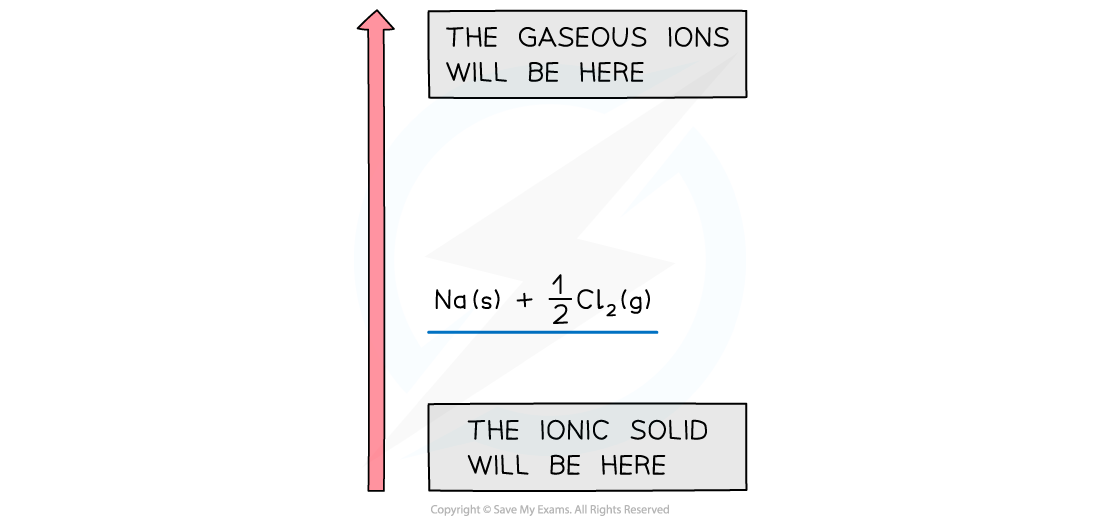Drawing a Born-Haber cycle step 1

• Next, we need to create the gaseous ions
• This is a two step process of first creating the gaseous atoms and then turning them into ions
• Creating gaseous atoms is a bond breaking process, so arrows must be drawn upwards
• It doesn't matter whether you start with sodium or chlorine
• The enthalpy of atomisation of sodium is

Na (s) → Na (g)           ΔHatꝋ = +108 kJ mol -1

• The enthalpy of atomisation of chlorine is

½Cl2 (g) → Cl (g)       ΔHatꝋ = +121 kJ mol -1

• We can show the products of the process on the horizontal lines and the energy value against a vertical arrow connecting the energy levels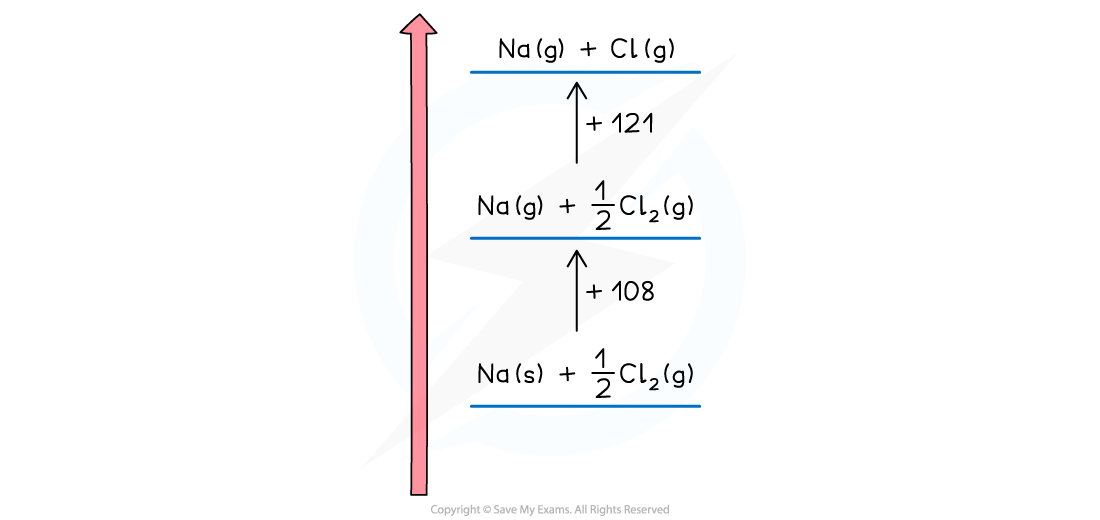Drawing a Born-Haber cycle step 2 - creating the gaseous atoms

• Now the ions are created
• The sodium ion loses an electron, so this energy change is the first ionisation energy for sodium

Na (g) → Na+ (g) + e–          ΔHieꝋ = +500 kJ mol-1

• The change is endothermic so the direction continues upwards
• The chlorine atom gains an electron, so this is electron affinity

Cl (g) + e– → Cl- (g)           ΔHeaꝋ = -364 kJ mol-1

• The exothermic change means this is downwards
• The change is displaced to the right to make the diagram easier to read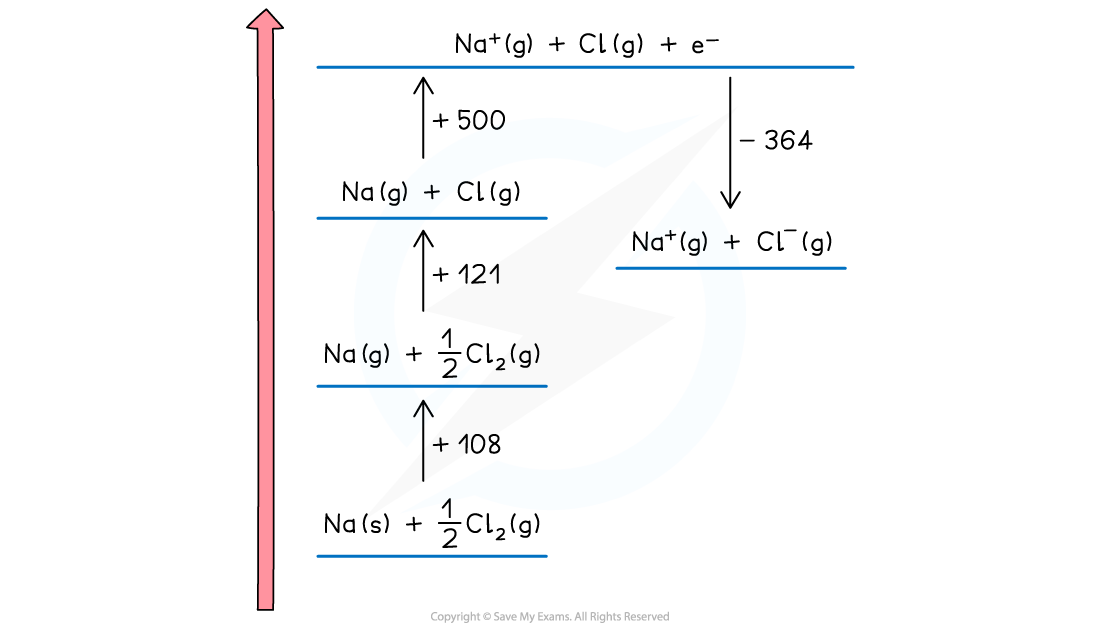Drawing a Born-Haber cycle step 3 - creating the gaseous ions

• The two remaining parts of the cycle can now be completed
• The enthalpy of formation of sodium chloride is added at the bottom of the diagram

Na (s) + ½Cl2 (g) → NaCl (s)            ΔHfꝋ = -411 kJ mol -1

• This is an exothermic change for sodium chloride so the arrow points downwards
• Enthalpy of formation can be exothermic or endothermic, so you may need to show it above the elements ( and displaced to the right) for a endothermic change
• The final change is lattice enthalpy, which is usually shown a formation. For sodium chloride the equation is

Na+(g) + Cl-(g) → NaCl (s)  ΔHlattꝋ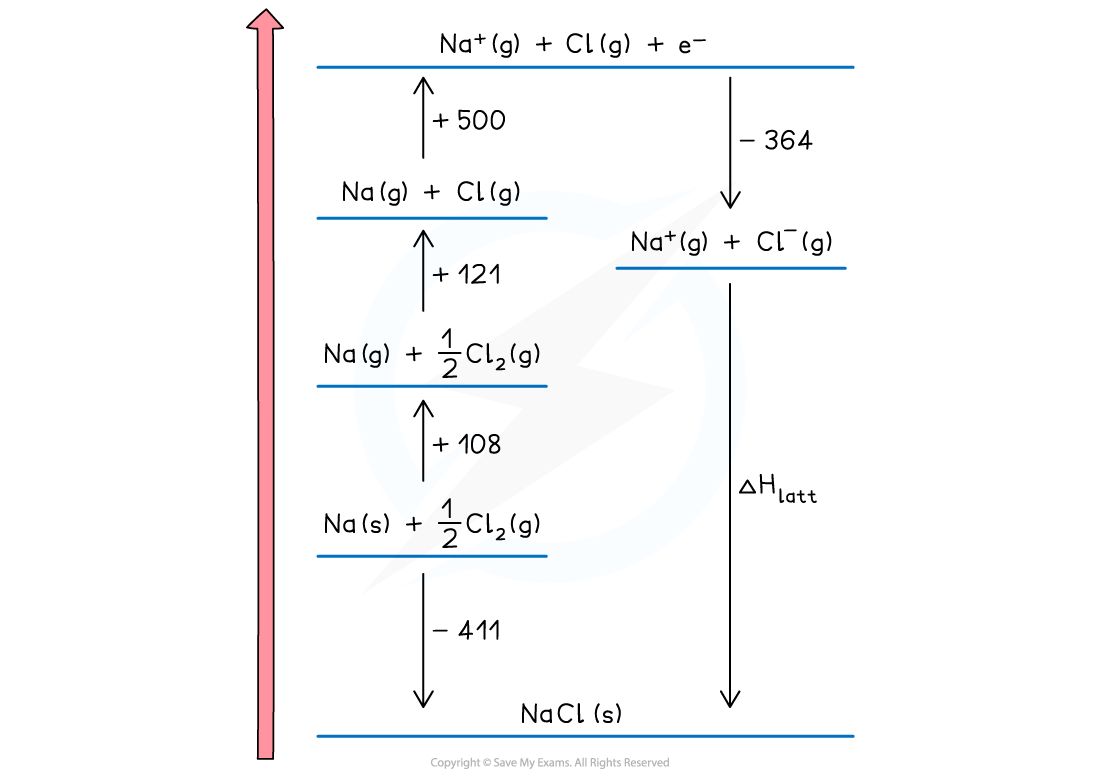Drawing a Born-Haber cycle step 4 - completing the cycle

• The cycle is now complete
• The cycle is usually used to calculate the lattice enthalpy of an ionic solid, but can be used to find other enthalpy changes if you are given the lattice enthalpy

#### Worked Example

Constructing a Born-Haber cycle for KCl

Construct a Born-Haber Cycle which can be used to calculate the lattice energy of potassium chloride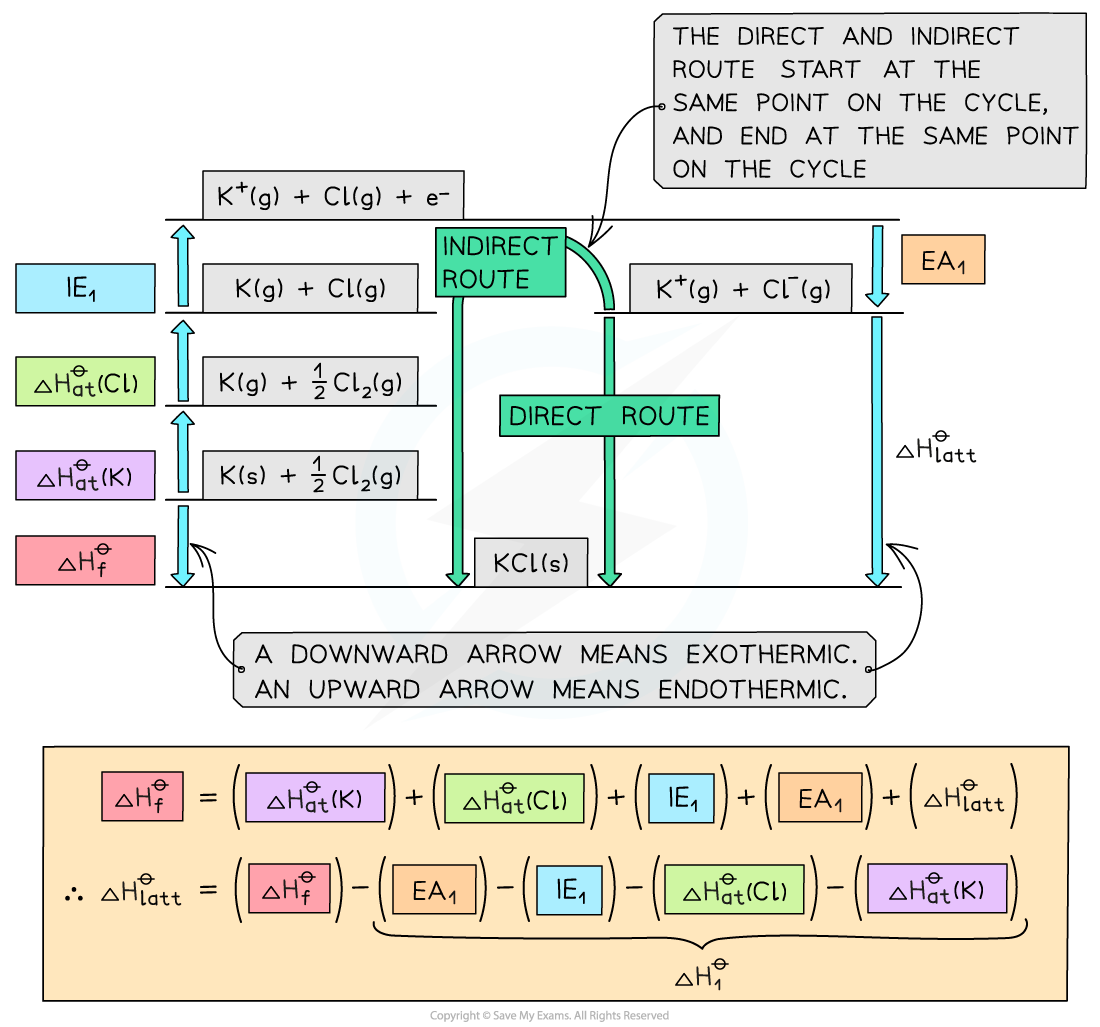#### Worked Example

Constructing a Born-Haber cycle for MgO

Construct a Born-Haber Cycle which can be used to calculate the lattice energy of magnesium oxide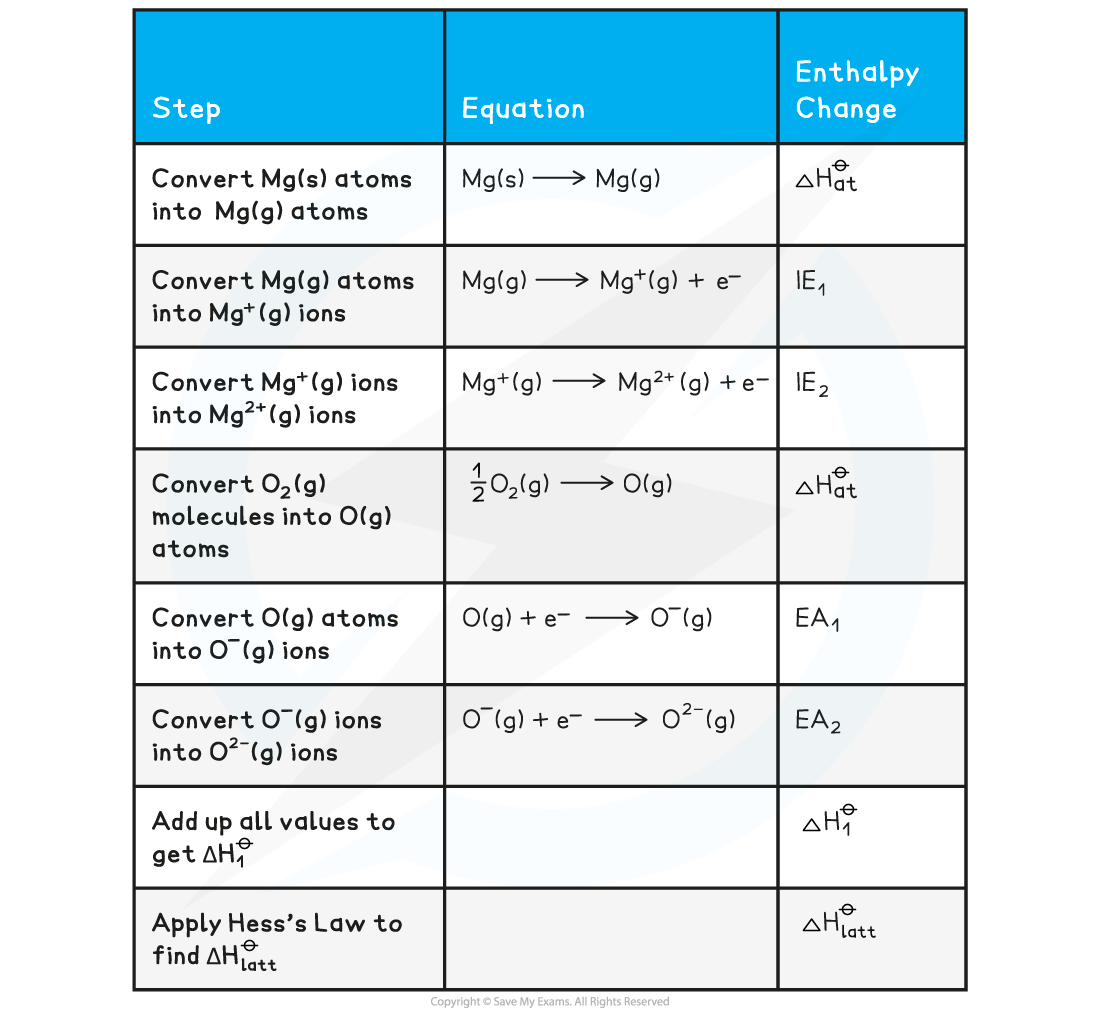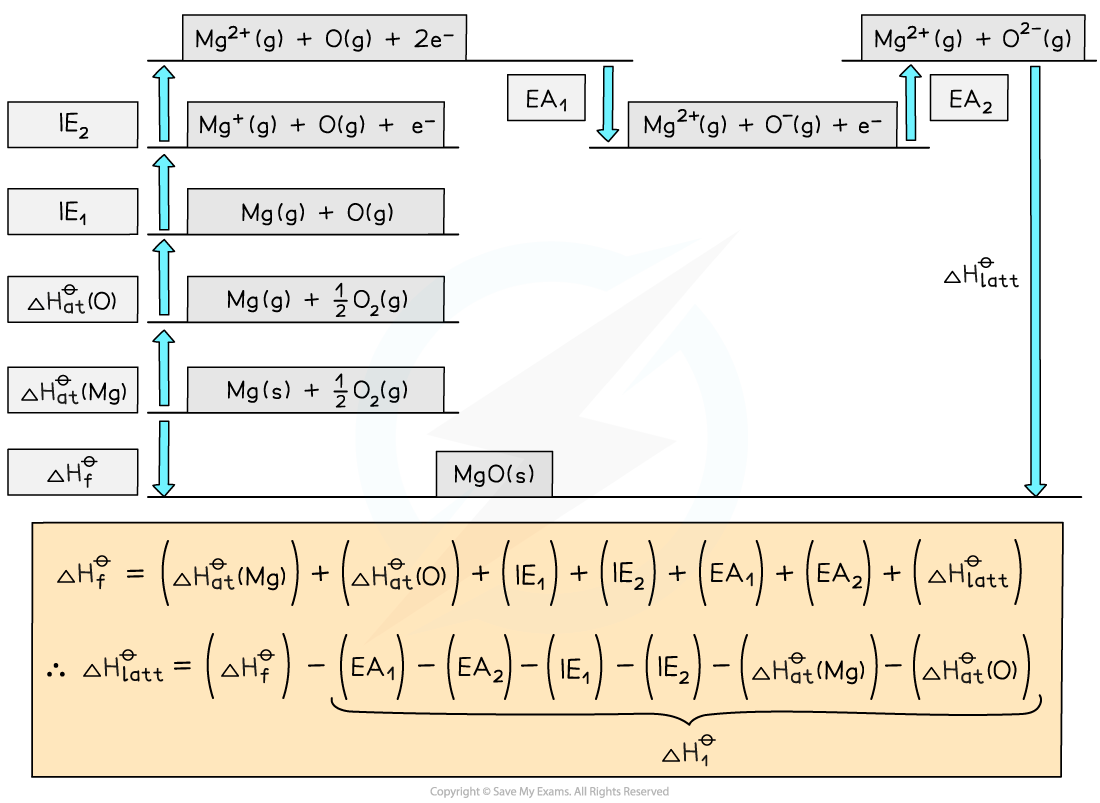• Once a Born-Haber cycle has been constructed, it is possible to calculate the lattice energy (ΔHlattꝋ) by applying Hess’s law and rearranging:

ΔHfꝋ = ΔHatꝋ + ΔHatꝋ + IE + EA + ΔHlattꝋ

• If we simplify this into three terms, this makes the equation easier to see:
• ΔHlattꝋ
• ΔHfꝋ
• ΔH1ꝋ (the sum of all of the various enthalpy changes necessary to convert the elements in their standard states to gaseous ions)

• The simplified equation becomes

ΔHfꝋ = ΔH1ꝋ + ΔHlattꝋ

So, if we rearrange to calculate the lattice energy, the equation becomes

ΔHlattꝋ = ΔHfꝋ - ΔH1ꝋ

• When calculating the ΔHlattꝋ, all other necessary values will be given in the question
• A Born-Haber cycle could be used to calculate any stage in the cycle
• For example, you could be given the lattice energy and asked to calculate the enthalpy change of formation of the ionic compound
• The principle would be exactly the same
• Work out the direct and indirect route of the cycle (the stage that you are being asked to calculate will always be the direct route)
• Write out the equation in terms of enthalpy changes and rearrange if necessary to calculate the required value

• Remember: sometimes a value may need to be doubled or halved, depending on the ionic solid involved
• For example, with MgCl2 the value for the first electron affinity of chlorine would need to be doubled in the calculation, because there are two moles of chlorine atoms
• Therefore, you are adding 2 moles of electrons to 2 moles of chlorine atoms, to form 2 moles of Cl- ions

#### Worked Example

Calculating the lattice energy of KCl

Given the data below, calculate the ΔHlattꝋ of potassium chloride (KCl)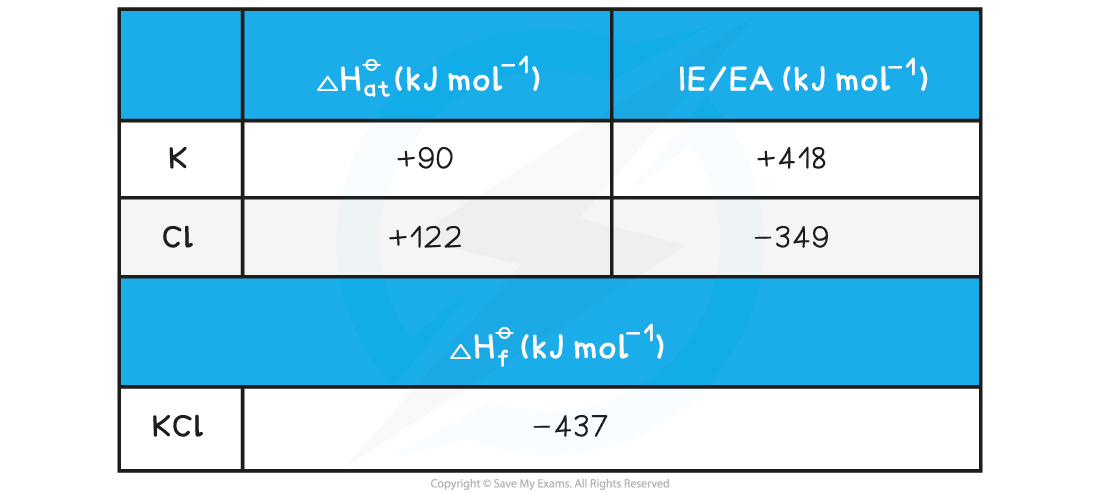Step 1: The corresponding Born-Haber cycle is:Step 2: Applying Hess’ law, the lattice energy of KCl is:

ΔHlattꝋ = ΔHfꝋ - ΔH1ꝋ

ΔHlattꝋ = ΔHfꝋ - [(ΔHatꝋ K) + (ΔHatꝋ Cl) + (IE1 K) + (EA1 Cl)]

Step 3: Substitute in the numbers:

ΔHlattꝋ = (-437) - [(+90) + (+122) + (+418) + (-349)] = -718 kJ mol-1

#### Worked Example

Calculating the lattice energy of MgO

Given the data below, calculate the of ΔHlattꝋ magnesium oxide of magnesium oxide (MgO)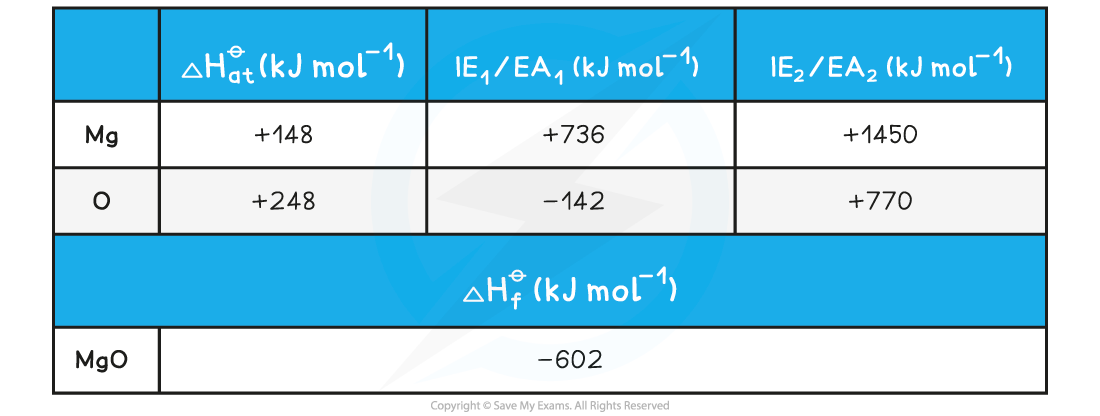Step 1: The corresponding Born-Haber cycle is:Step 2: Applying Hess’ law, the lattice energy of MgO is:

ΔHlattꝋ = ΔHfꝋ - ΔH1ꝋ

ΔHlattꝋ = ΔHfꝋ - [(ΔHatꝋ Mg) + (ΔHatꝋ O) + (IE1 Mg) + (IE2 Mg) + (EA1 O) + (EA2 O)]

Step 3: Substitute in the numbers:

ΔHlattꝋ = (-602) - [(+148) + (+248) + (+736) + (+1450) + (-142) + (+770)]

= -3812 kJ mol-1